# Presse universelle MecaMotion par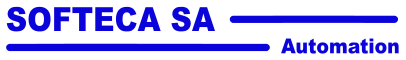Navigation: Part program instructions > Controls "Stop on force" control instruction

"Stop on force" control instruction

Unlike the "stop on signal" instruction, which stops the axis as soon as a signal passes a certain threshold, the "stop on force" control is dedicated to stopping the axis in relation to the force, with a regulation of the feed velocity in order to have, a final force very close to the setpoint given.

The regulator incorporated in this control is of type "P" (proportional). With the gain adjustable directly in the instruction.

This regulator works as follows:

Every 2[ms] the value of the force sensor is read and compared with the setpoint.

As the difference between the setpoint and the measured force decreases, the axis velocity will decrease (proportionally) until it reaches the minimum velocity (parameter "minimal velocity").

The regulator will start to operate as soon as the axis reaches the working position. At this point, the velocity will decrease by "X"[%] of its previous velocity (parameter "working velocity [%]") and after that, the velocity will be regulated.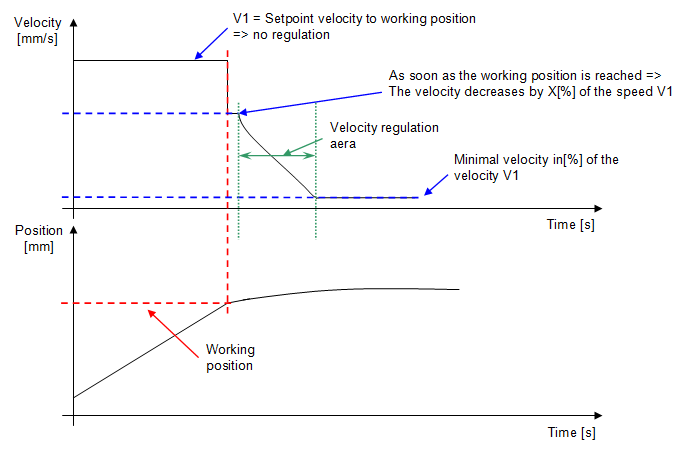Figure 1: Graphical explanation of how the "stop on force" control works

Below is a list of the instruction's input and output parameters:

Parameter name

Declaration

Type of data

Default value

Description

Working position [mm]

Input

LREAL

0[mm]

Position from which the feed velocity will first be reduced to X[%] of its initial value and then regulated

Force setpoint [N]

Input

LREAL

10[N]

Force setpoint to be reached

Velocity regulator gain [-]

Input

LREAL

1

Proportional gain of the regulator. The higher this value, the faster the approach velocity will decrease.

Minimal velocity [%]

Input

LREAL

10[%]

Minimum velocity that the regulation can reach, the velocity cannot go below this value. This one is given as a percentage of the initial axis velocity.

Working velocity [%]

Input

LREAL

50[%]

Percentage decrease in velocity from the initial velocity when the working position is reached.

Table 1: Summary of the different parameters of the instruction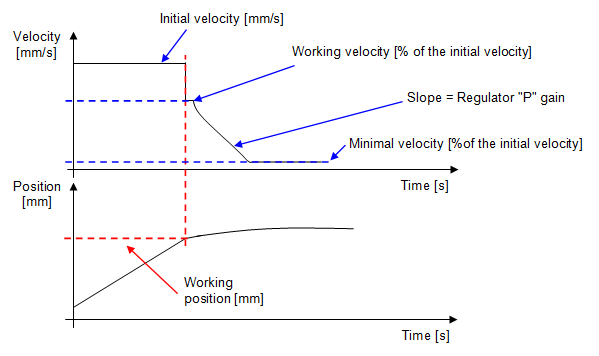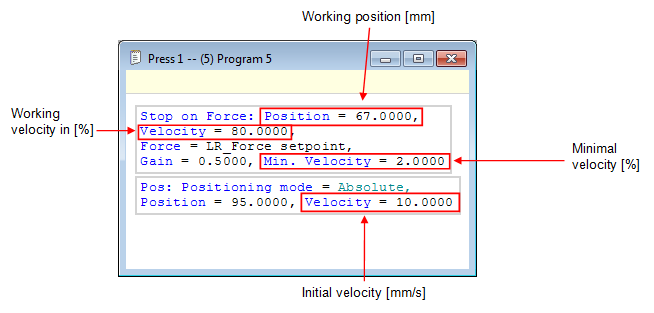Figure 2: Programming window and graphic explanation

Below you will find the calculation of the working velocity, the initial velocity is equal to 10[mm/s].

When switching to the working position, the axis velocity will change to:
Working velocity = 80[%] of 10[mm/s] = 8[mm/s] working velocity

The controller can reduce the axis velocity to:
Minimum velocity = 2[%] of 10[mm/s] = 0.2[mm/s]

 Example of use: We want to insert one part into another with a force of 150[N]. The final force must be as close as possible to the set point, which is why it is not possible to use the signal stop control, which is much less accurate. The gain, minimum velocity and working velocity parameters must be adjusted on test pieces, in order to be as precise as possible.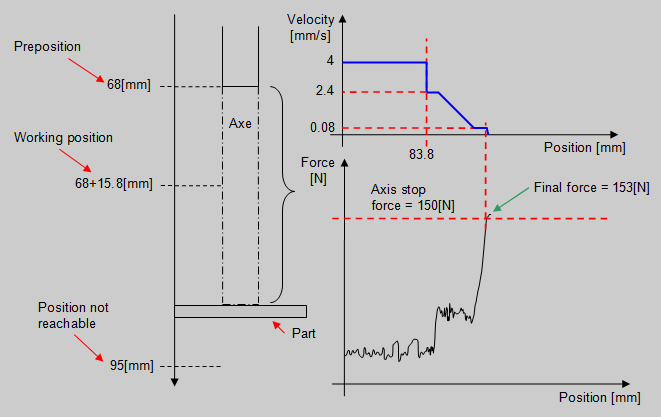Figure 3: Graphical explanation of the application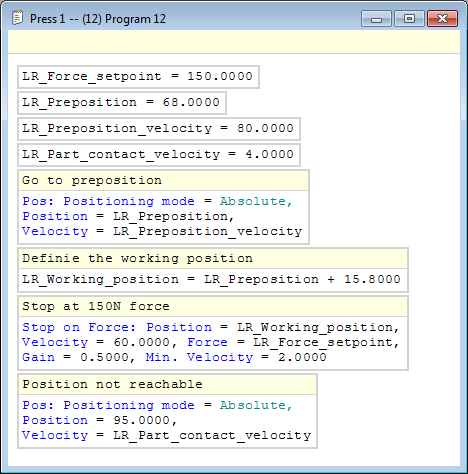Figure 4: Programming a "stop on force" instruction